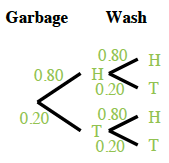### Home > CCG > Chapter 6 > Lesson 6.1.2 > Problem6-18

6-18.

Earl hates to take out the garbage and to wash the dishes, so he decided to make a deal with his parents: He will flip a coin once for each chore and will perform the chore if the coin lands on heads. What he doesn’t know is that his parents are going to use a weighted coin that lands on heads $80\%$ of the time!

1. What is the probability that Earl will have to do both chores?Garbage top edge left$\text{H }0.80$ top edge right$\text{T }0.20$ Wash left edge top$\text{H }0.80$ interior top left$\space$$0.64$$\space$ interior top right$\space$$0.16$$\space$ left edge bottom$\text{T }0.20$ interior bottom left blank$\space$$\space$$\space$ interior bottom right blank

What is the probability of flipping two heads in a row?

$(0.80)(0.80)=0.64=64\%$

2. What is the probability that Earl will have to take out the garbage, but will not need to wash the dishes?

Use a tree diagram or two-way table.

$(0.80)(0.20)=0.16=16\%$